# MSBSHSE Solutions For SSC (Class 10) Maths Part 1 Chapter 6 Statistics

MSBSHSE Solutions For SSC (Class 10) Maths Part 1 Chapter 6 Statistics is one of the best ways to strengthen one’s skills and knowledge. It contains all the relevant study material that can help the students score well in the examinations. Maharashtra Board Solutions Class 10 Chapter 6 Statistics is an important chapter from the examination perspective. A proper understanding of this chapter will further help the students with a few other chapters in the higher classes as well. The solutions are provided by the subject experts and are accurate. Every question is explained stepwise for a better understanding of the students. They can refer to these for reference purposes.

The best guide for the students is the Maharashtra Board Textbooks for Class 10 Maths Solutions. The students should go through the solutions after completing every chapter. This will help them analyse their weaknesses and improve on them. The PDF of Maharashtra Board Solutions for SSC Maths Chapter 6 Statistics is provided here. Students can refer and download from the given links. These solutions facilitate students to create good knowledge about the basic concepts of Mathematics. This chapter is based on mean, median and mode. Some of the essential topics of this chapter are listed below.

• Mean from classified frequency distribution
• Direct method
• Assumed mean method
• Step deviation method
• Median for grouped frequency distribution
• Mode for grouped frequency distribution
• Pictorial representation of statistical data
• Presentation of data
• Histogram
• Method of drawing a histogram
• Frequency polygon
• Pie diagram
• To draw a Pie diagram

## Download the PDF of Maharashtra Solutions For SSC Maths Part 1 Chapter 6 Statistics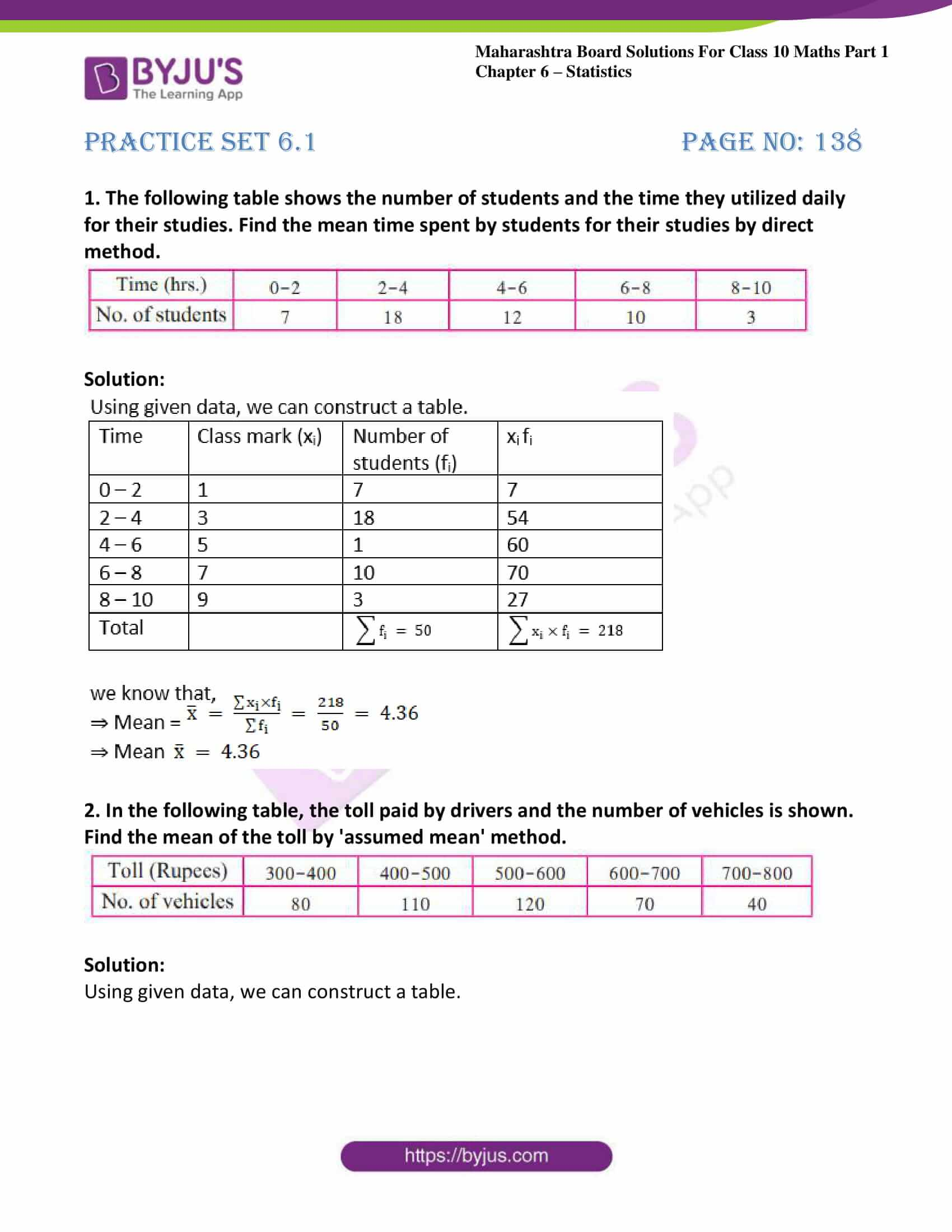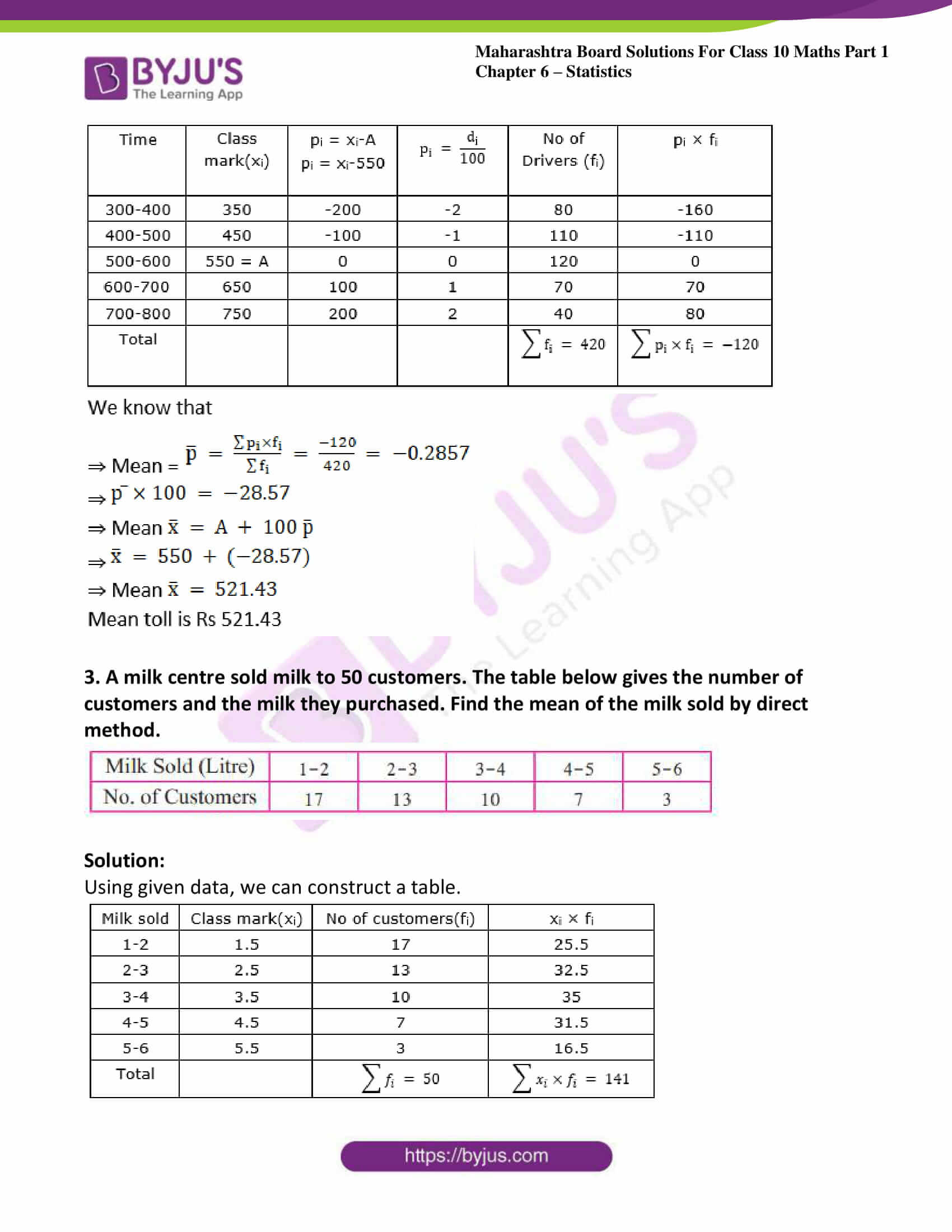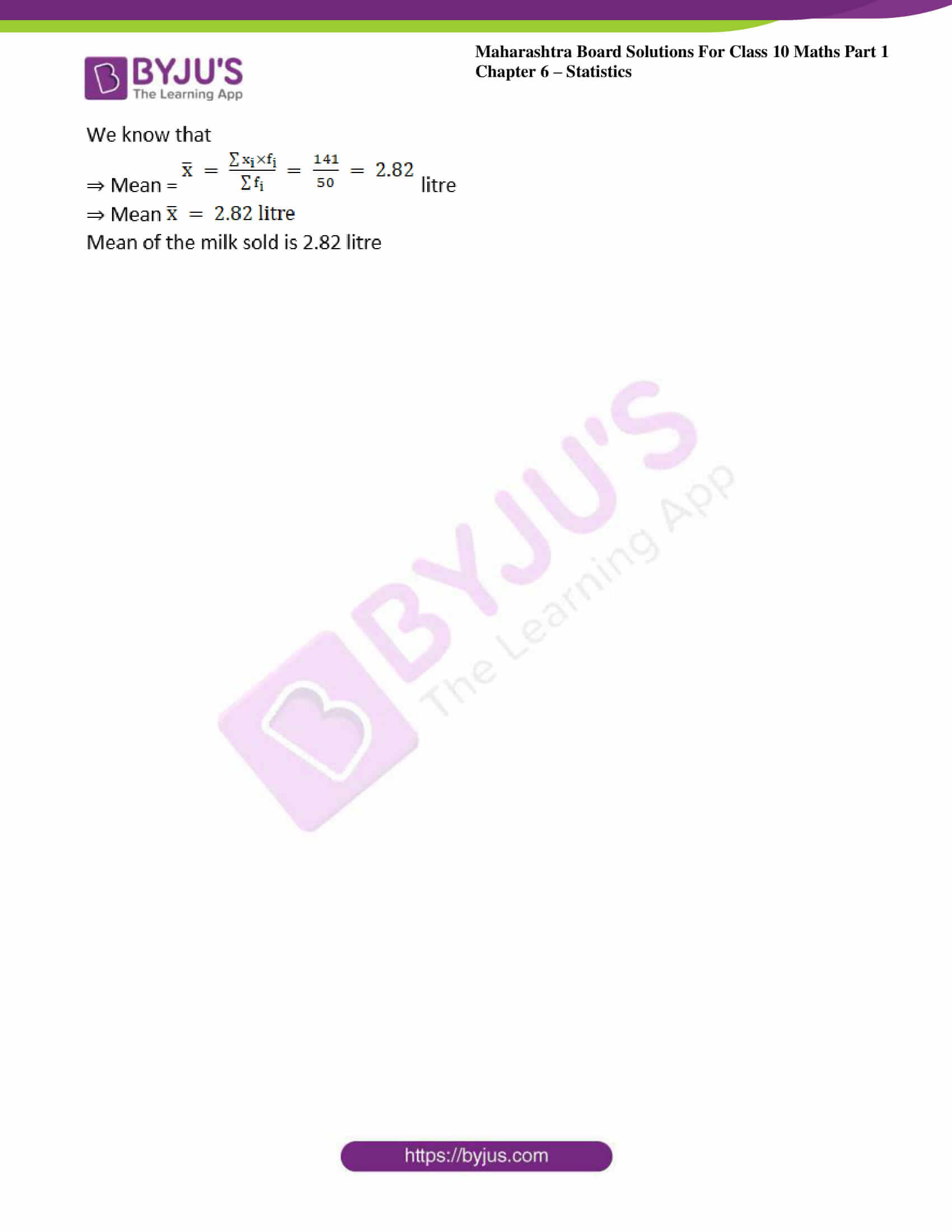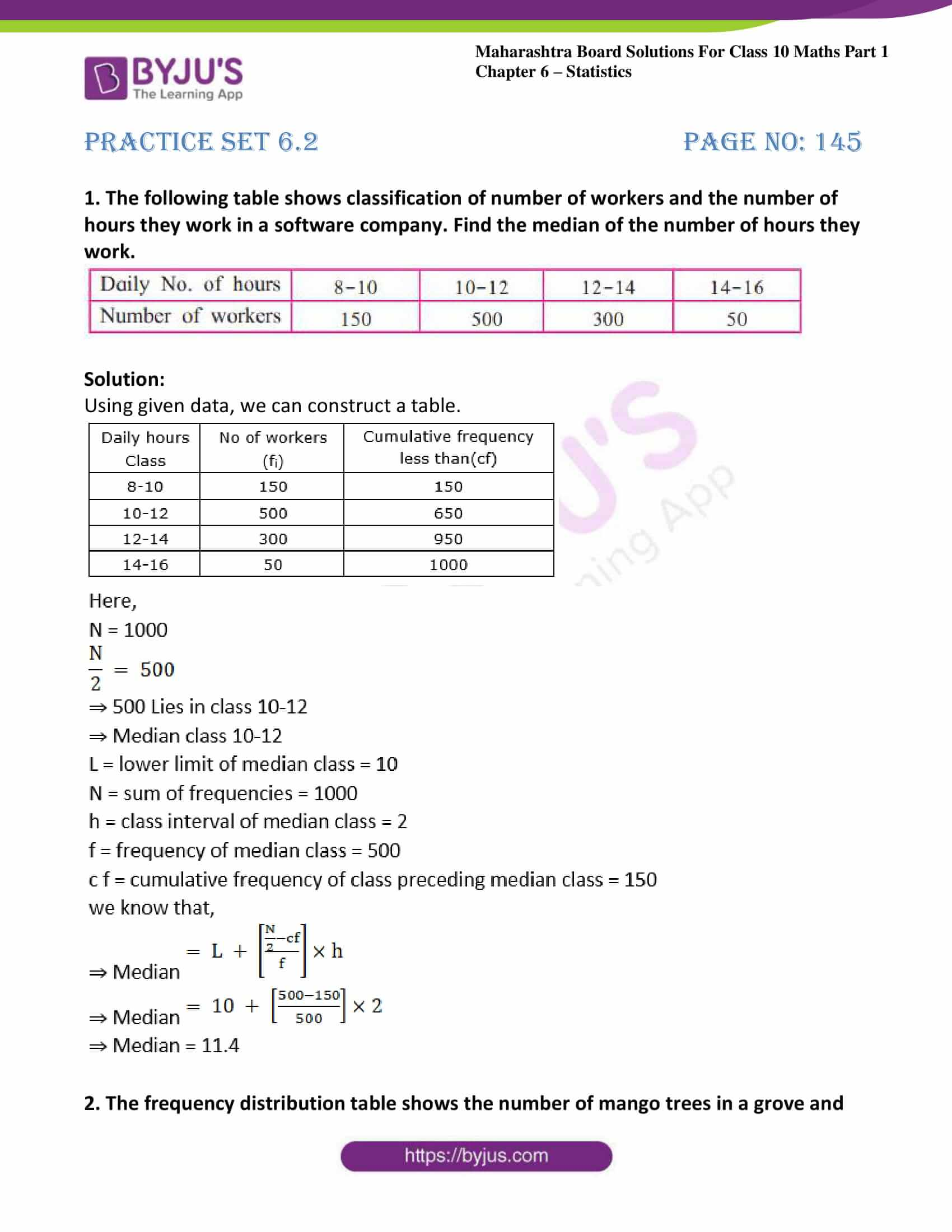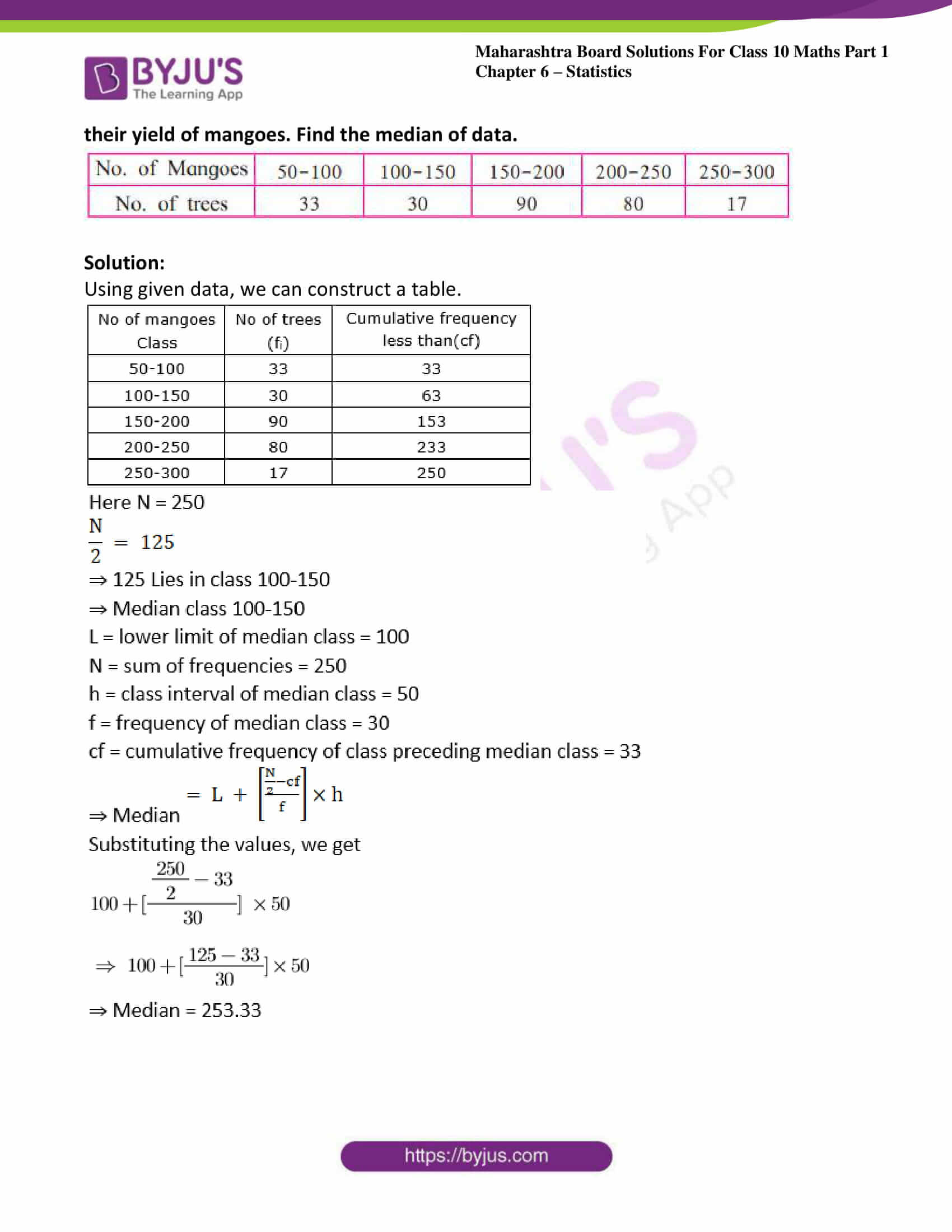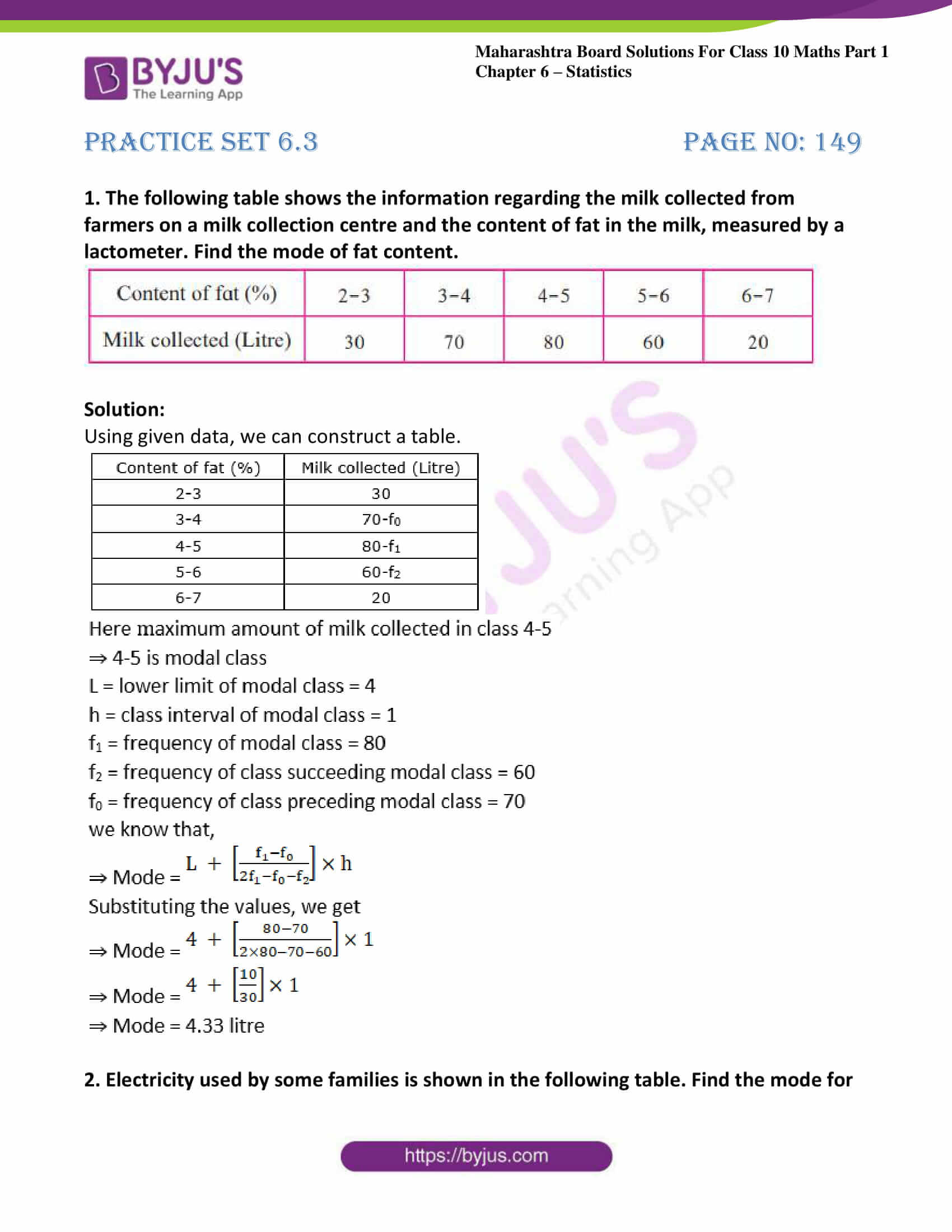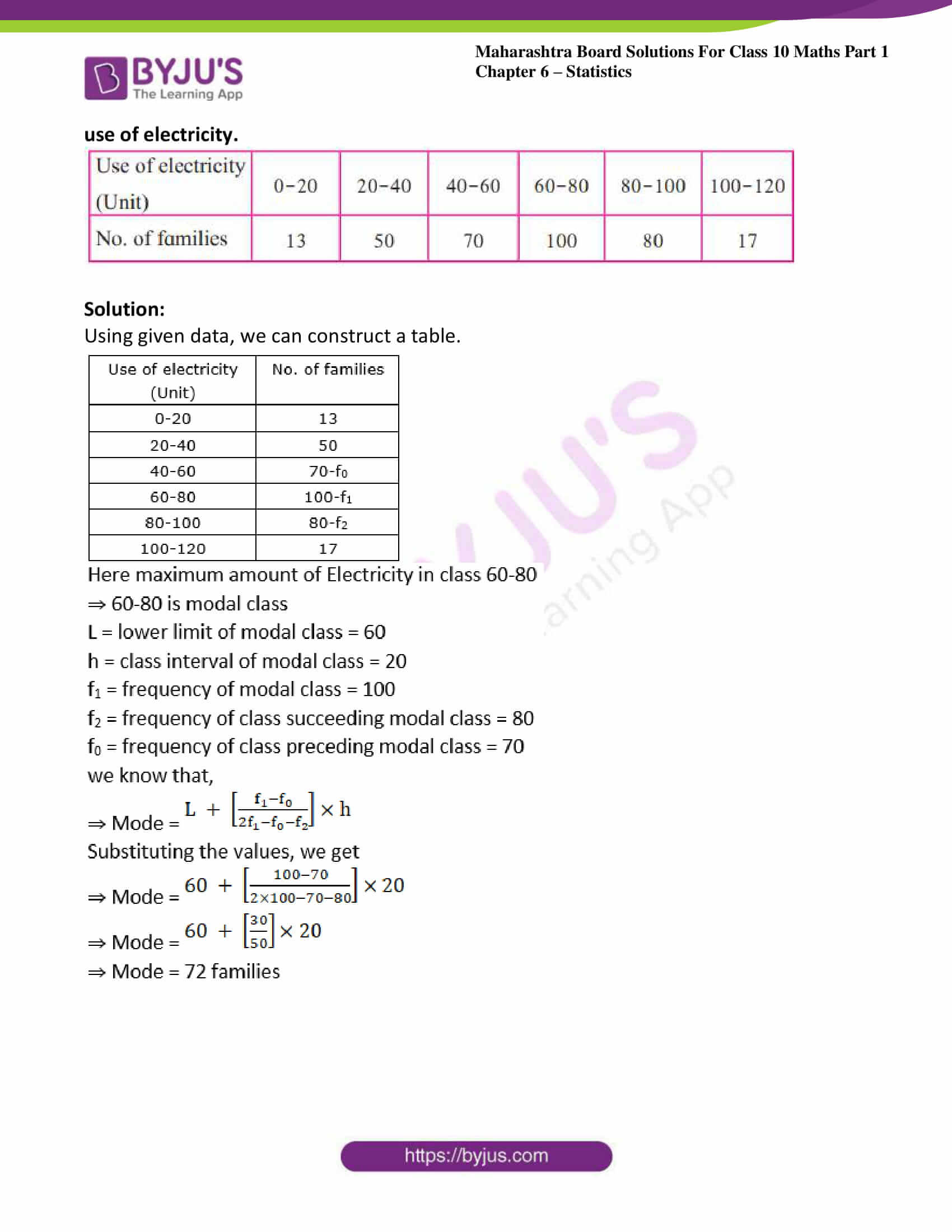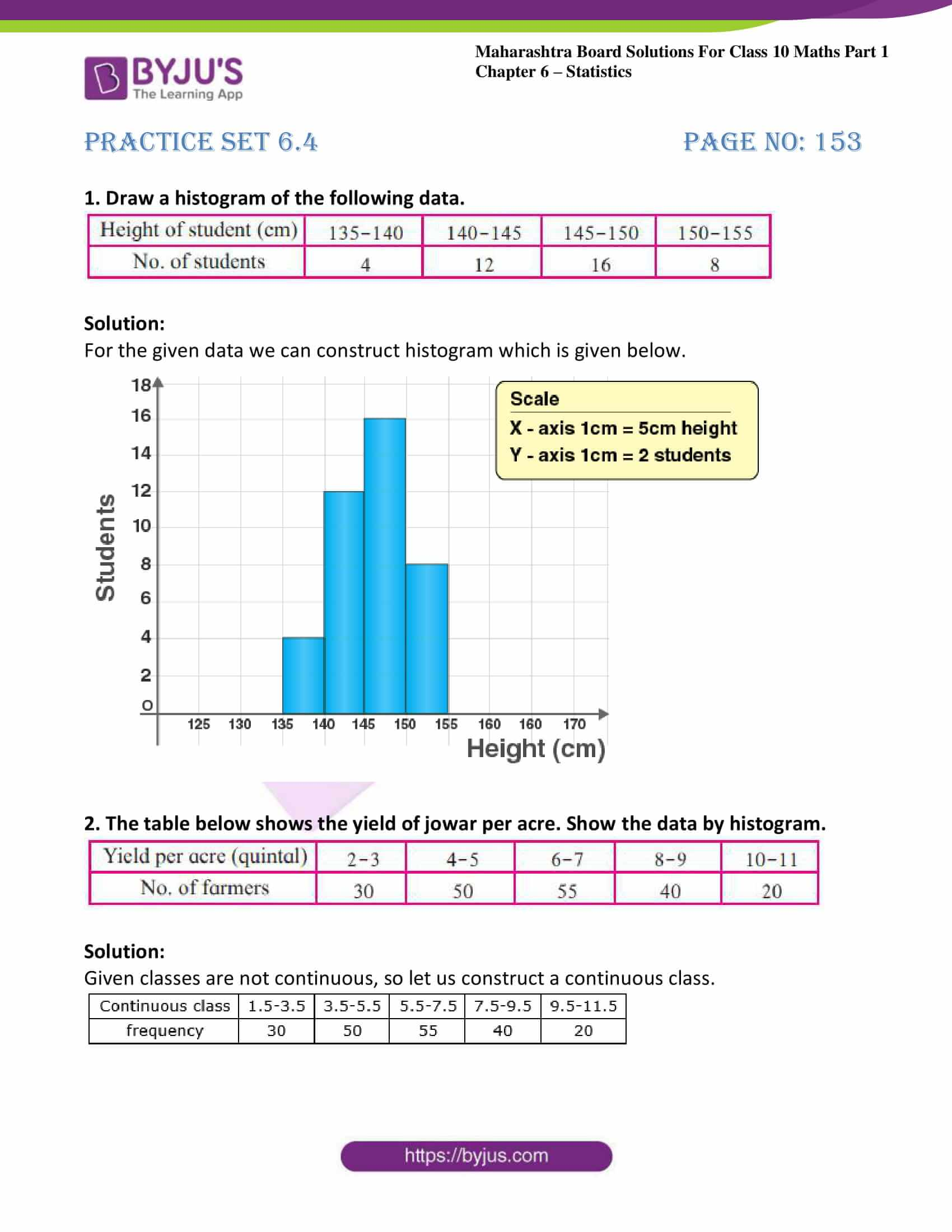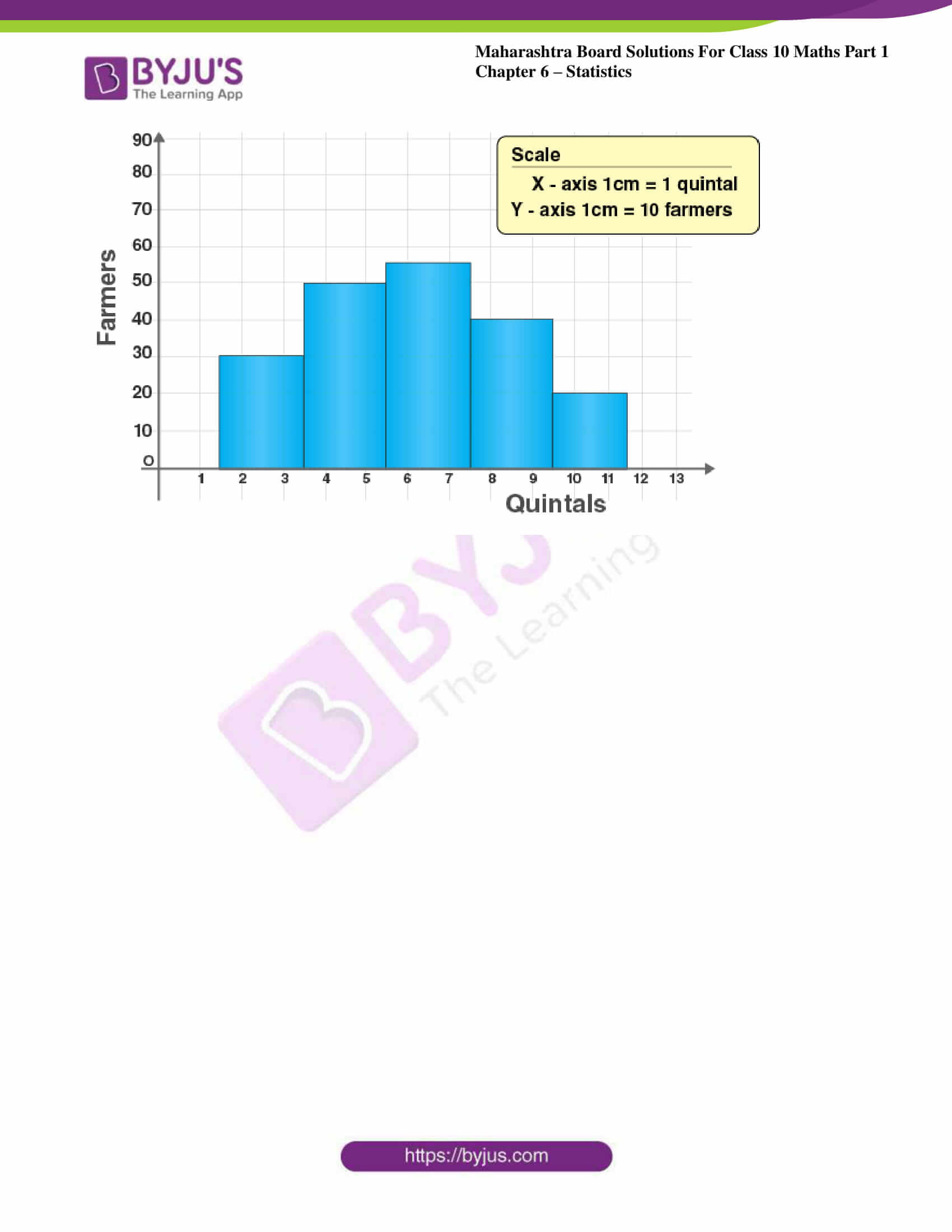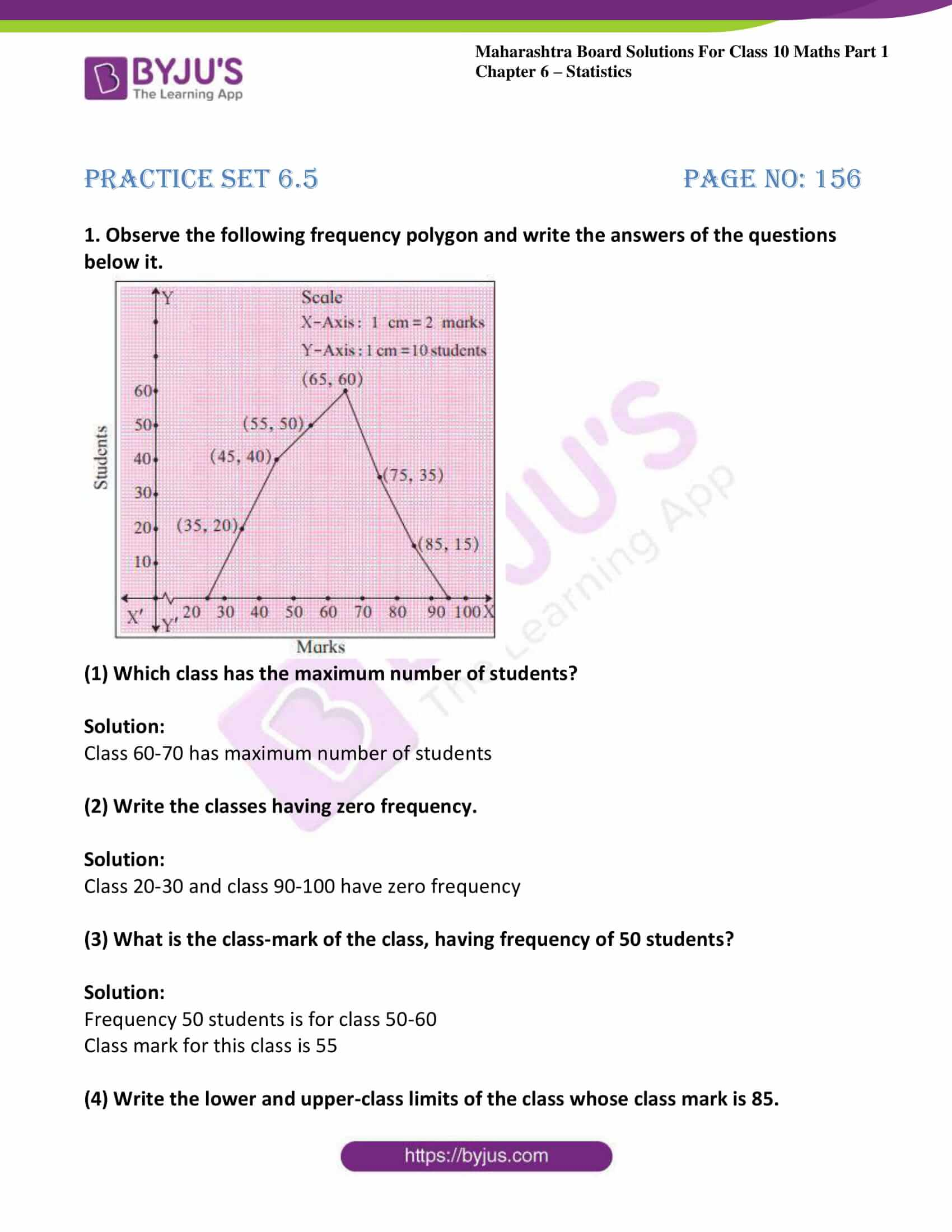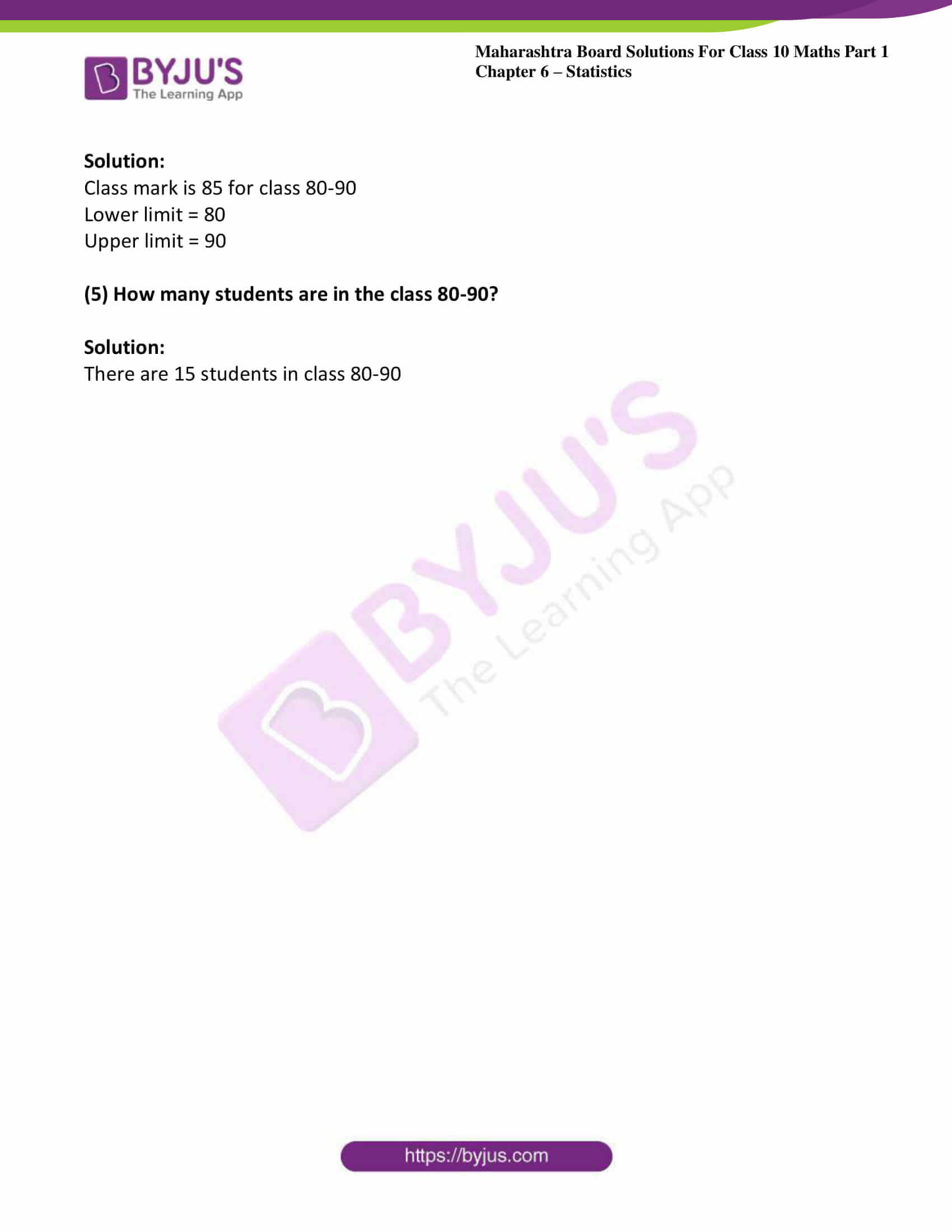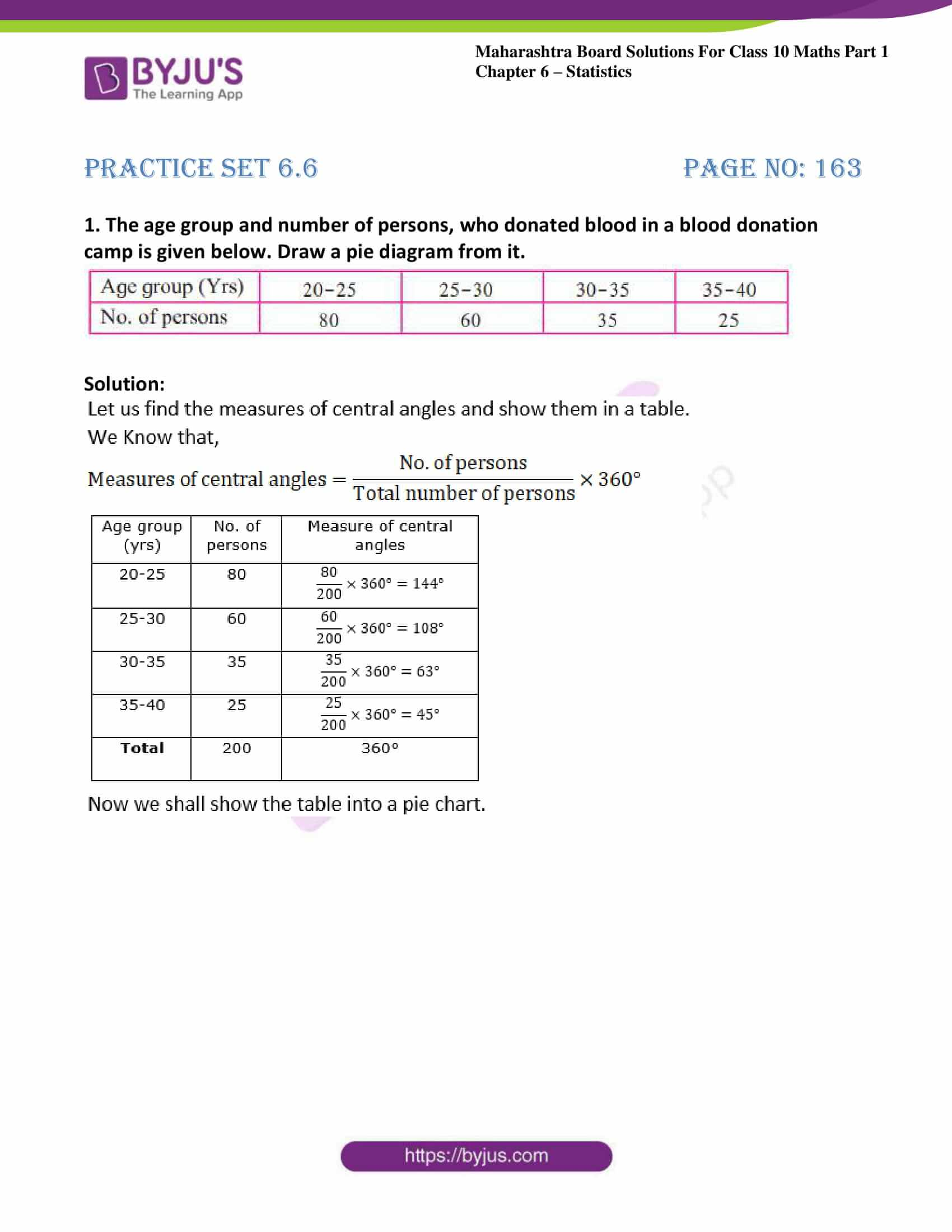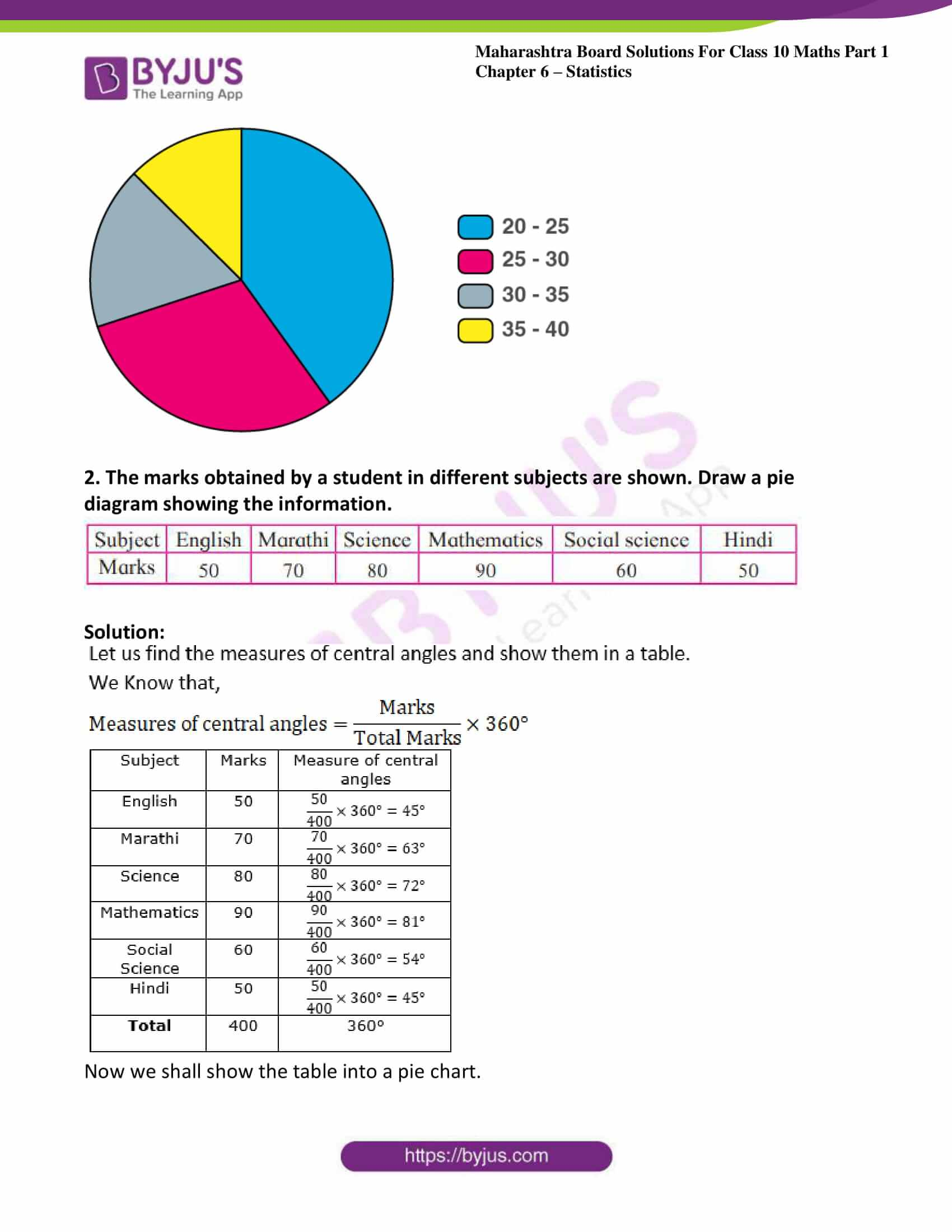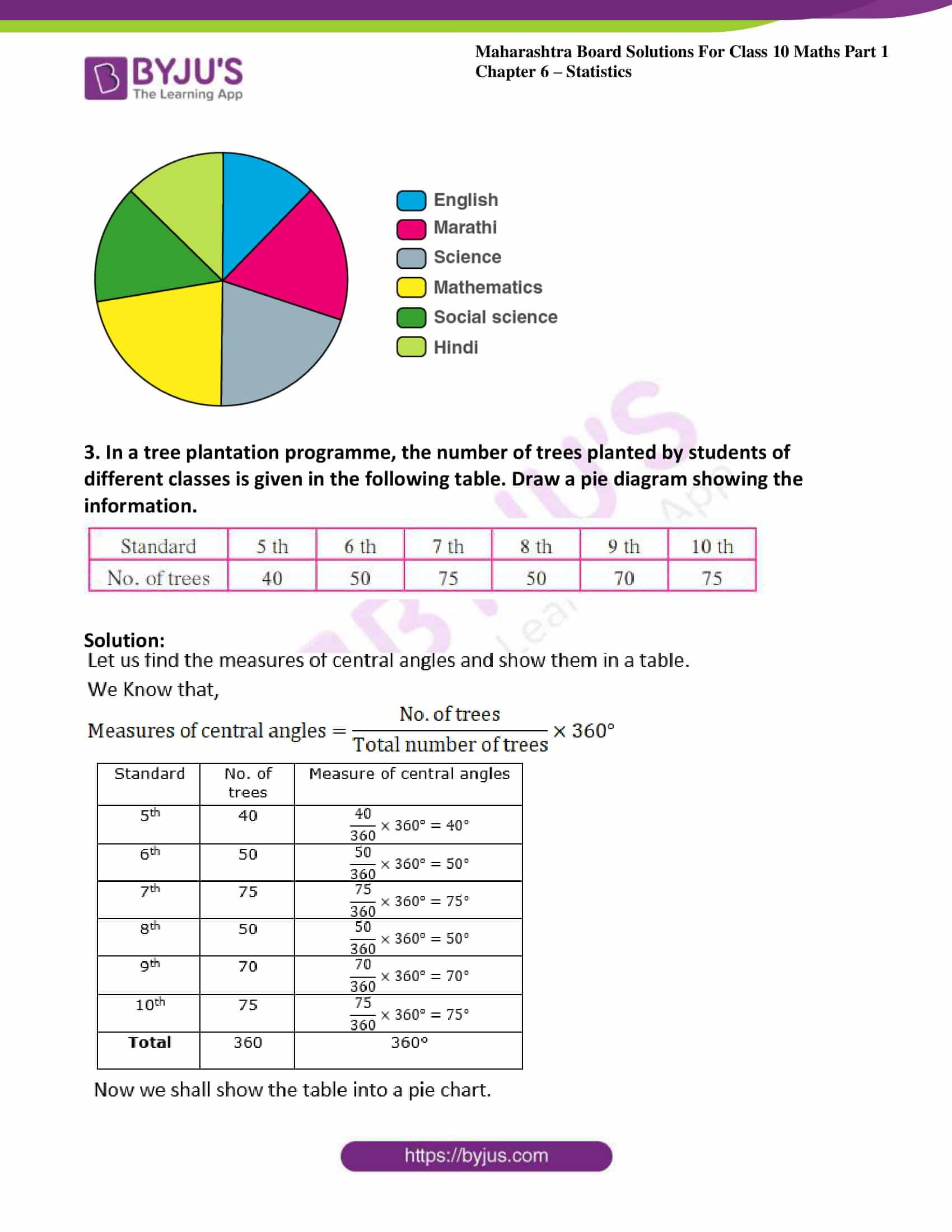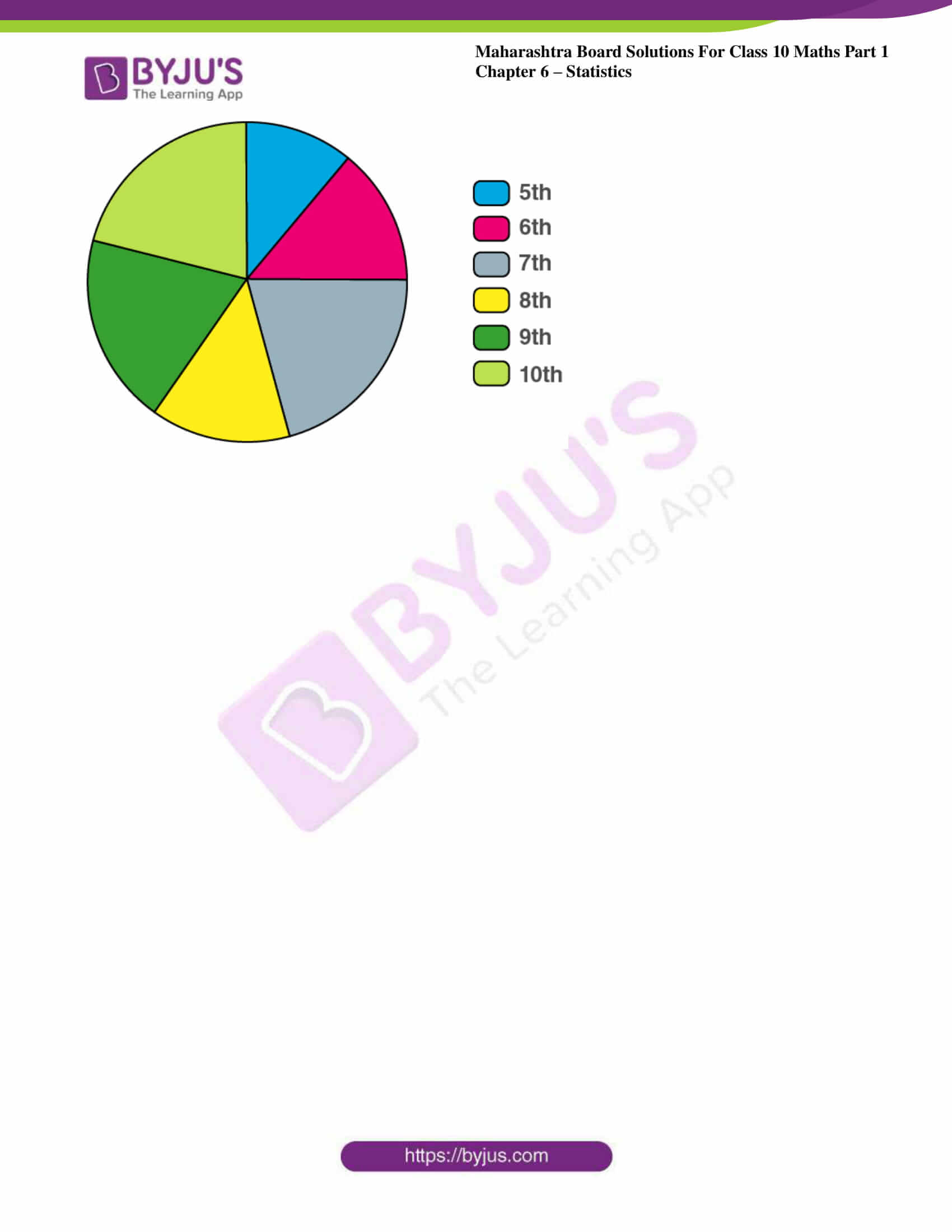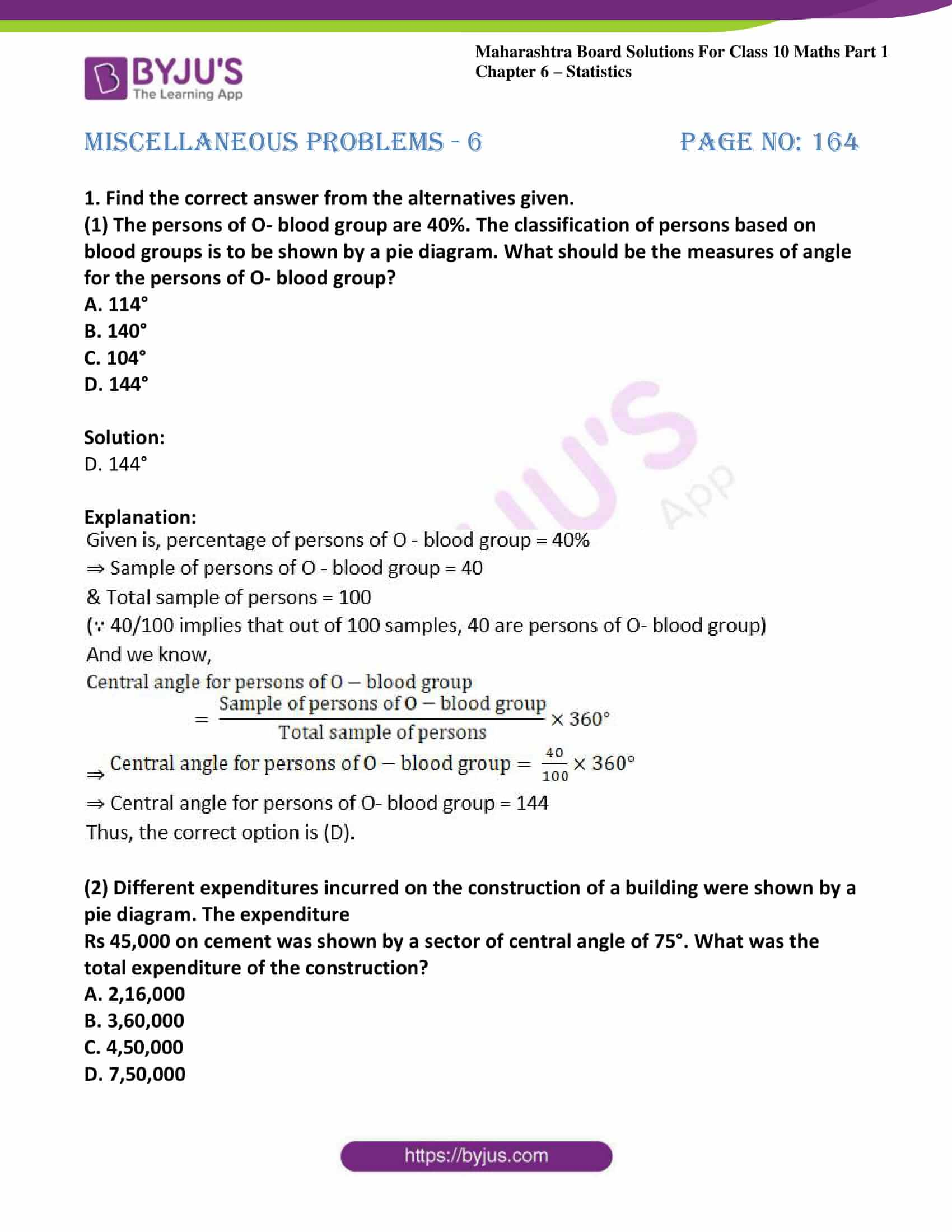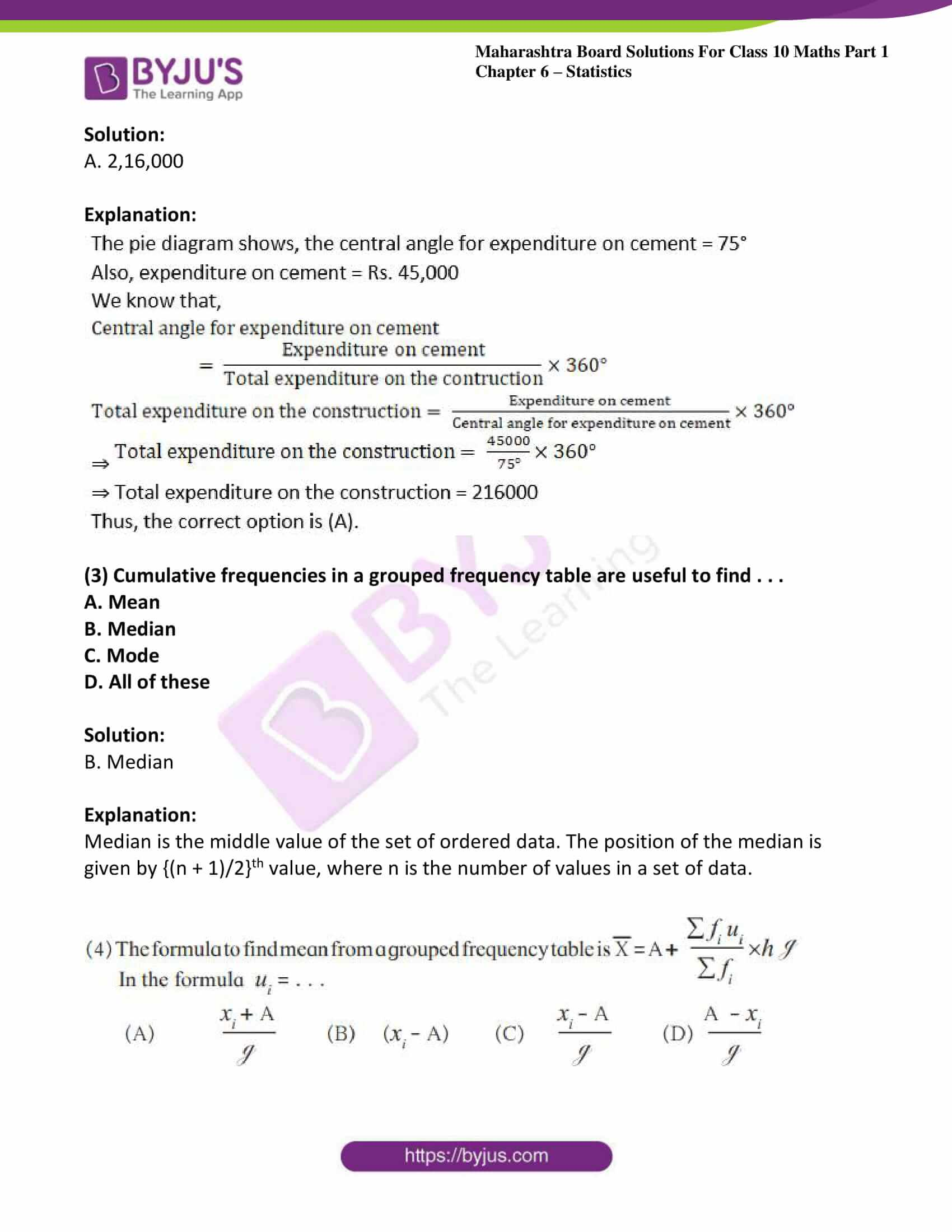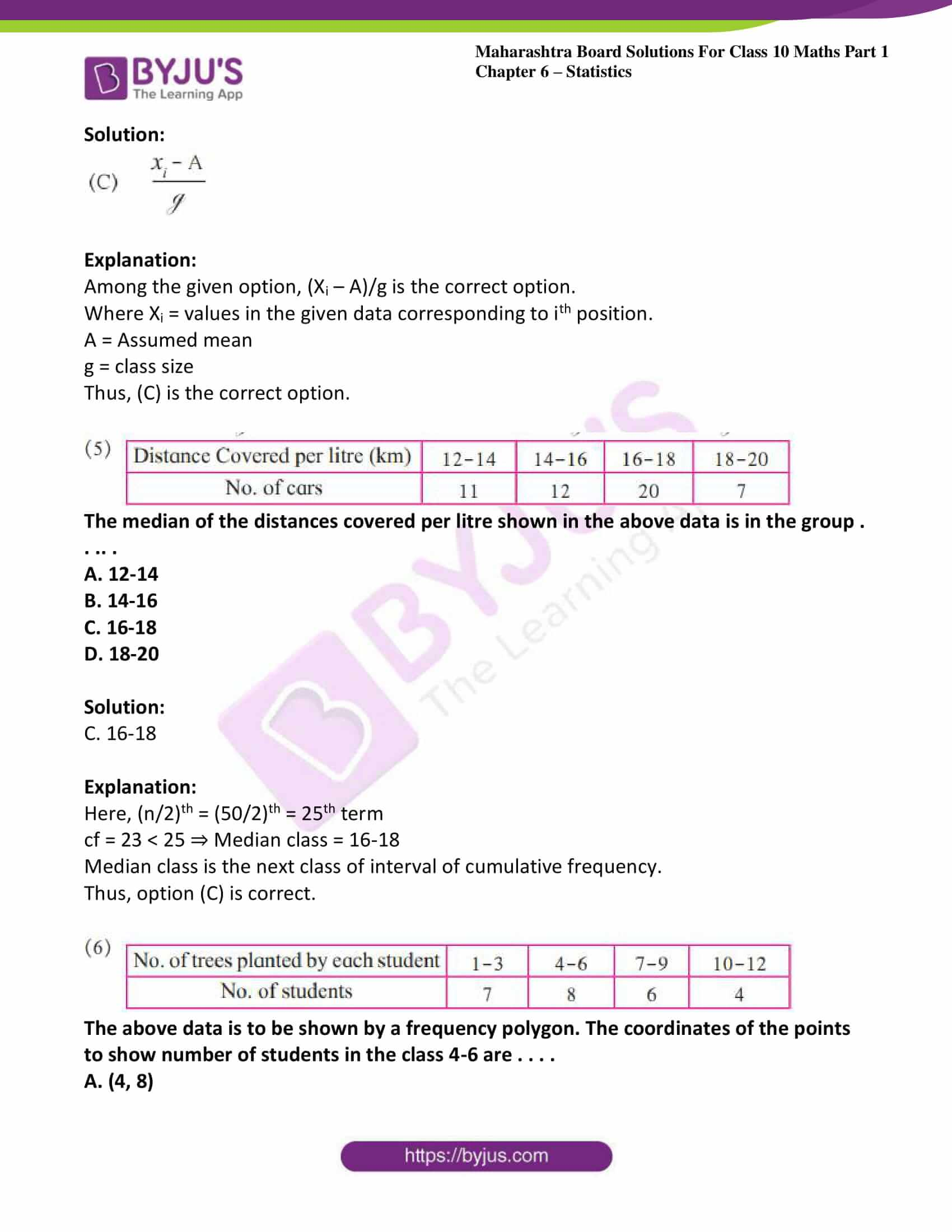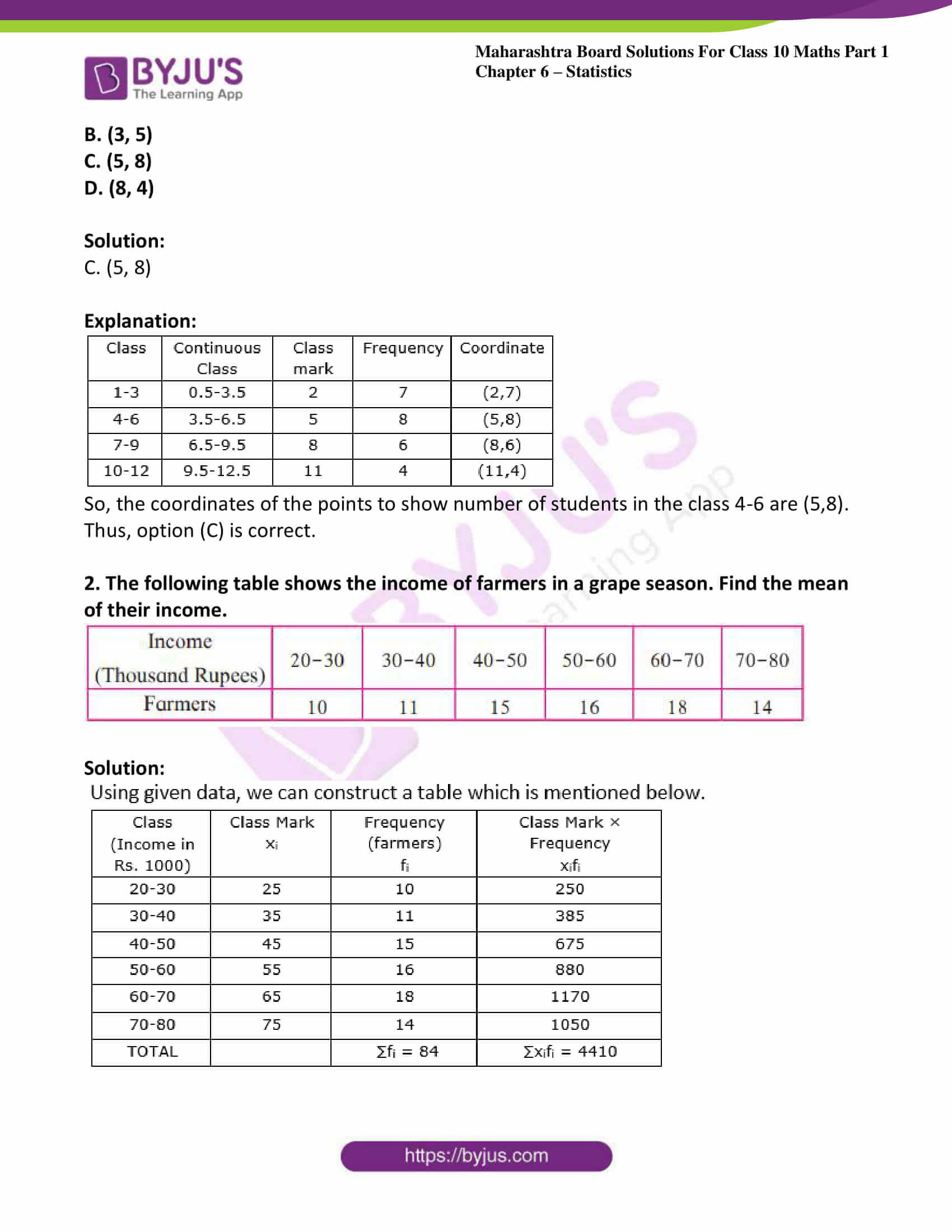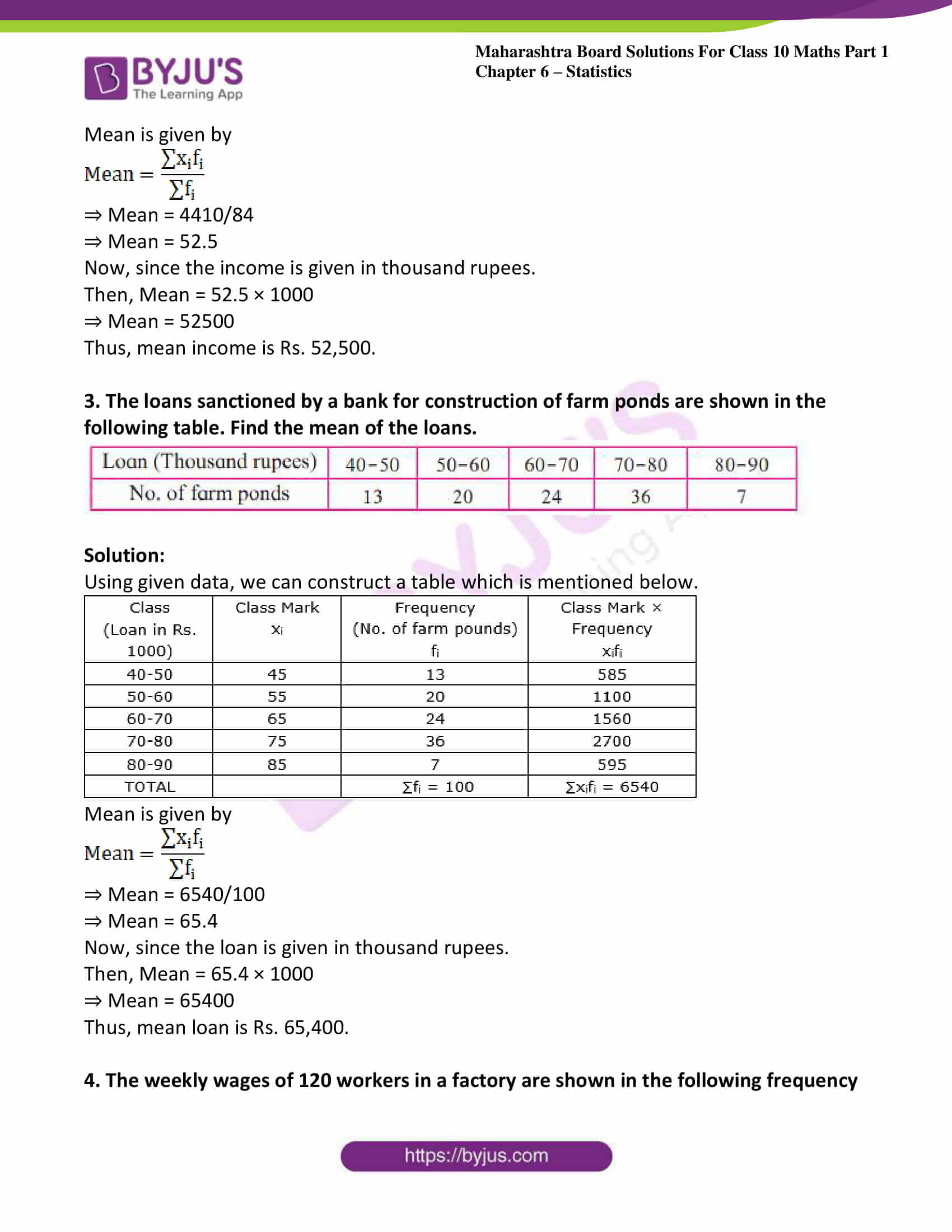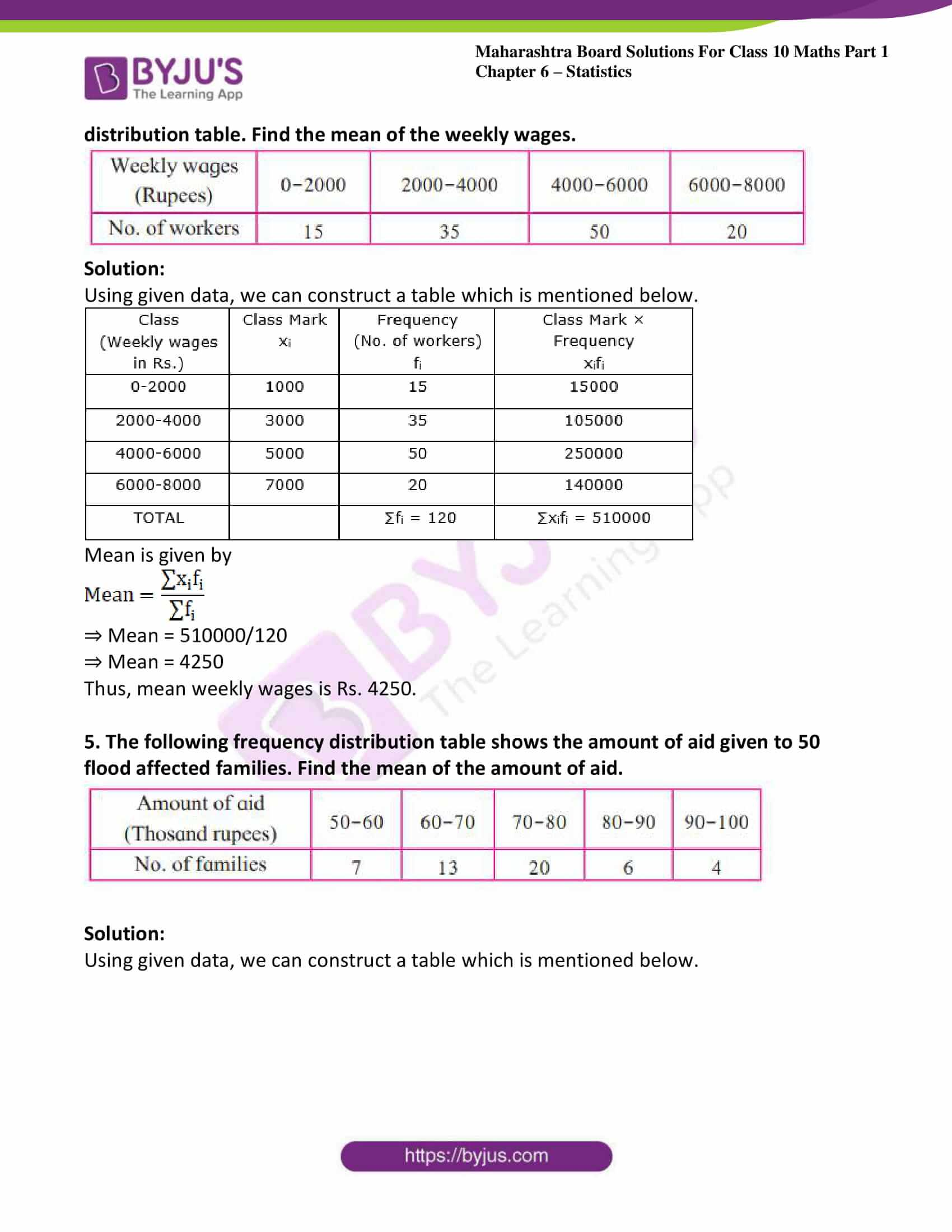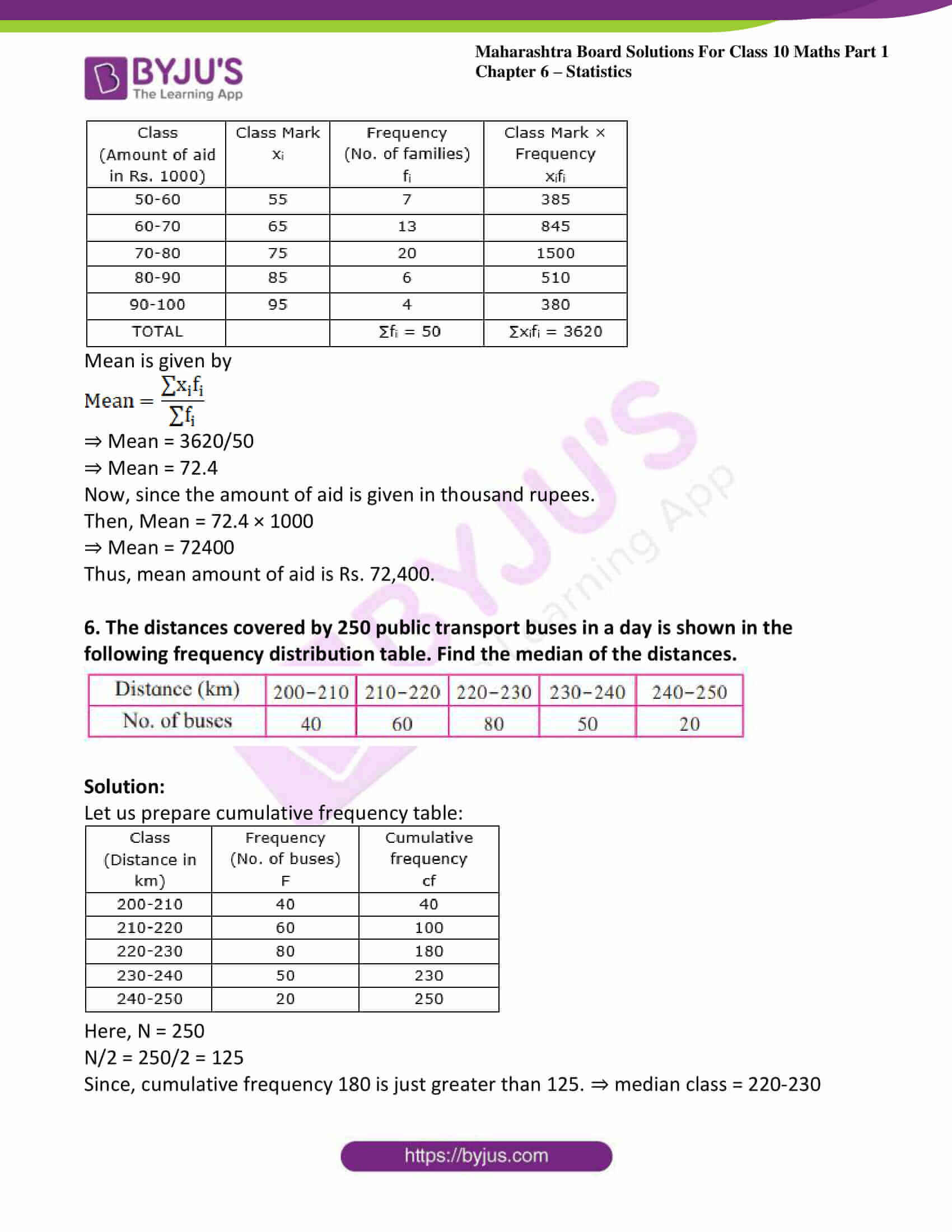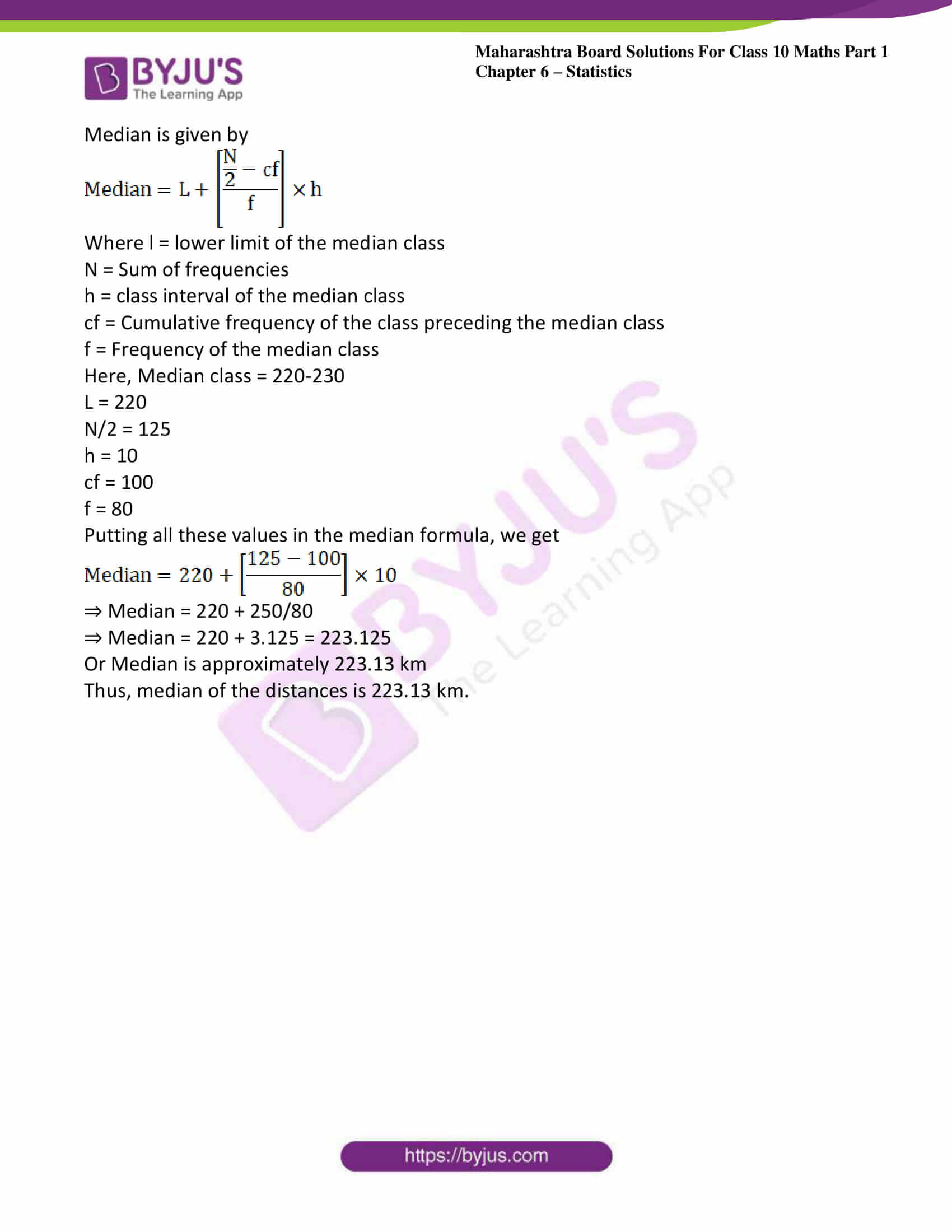### Access answers to Maths MSBSHSE Solutions For SSC Part 1 Chapter 6 – Statistics

Practice set 6.1 Page no: 138

1. The following table shows the number of students and the time they utilized daily for their studies. Find the mean time spent by students for their studies by direct method.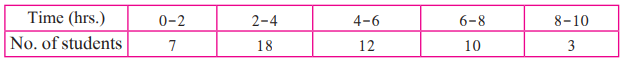Solution: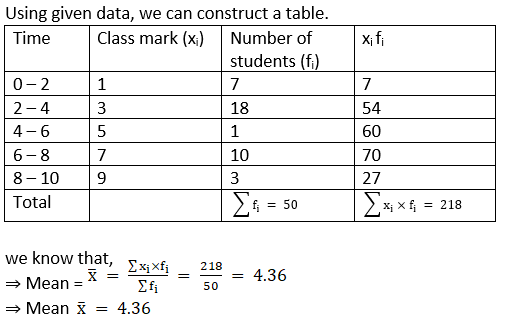2. In the following table, the toll paid by drivers and the number of vehicles is shown. Find the mean of the toll by ‘assumed mean’ method.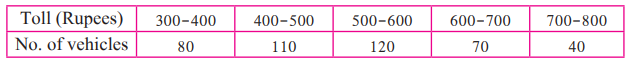Solution:

Using given data, we can construct a table.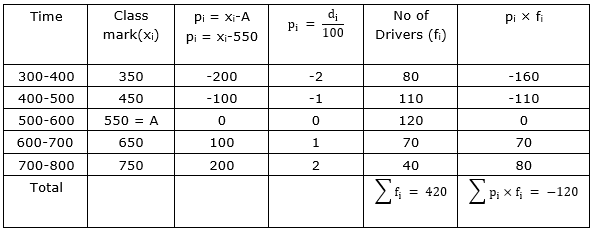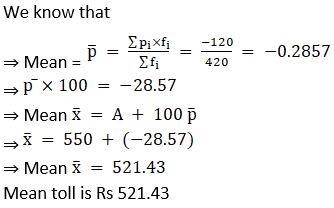3. A milk centre sold milk to 50 customers. The table below gives the number of customers and the milk they purchased. Find the mean of the milk sold by direct method.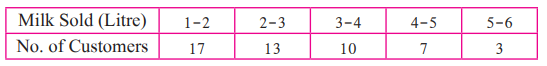Solution:

Using given data, we can construct a table.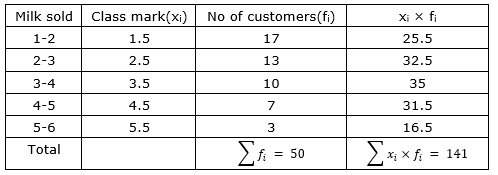Practice set 6.2 Page no: 145

1. The following table shows classification of number of workers and the number of hours they work in a software company. Find the median of the number of hours they work.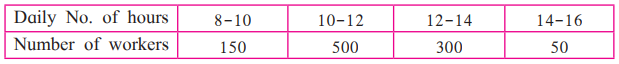Solution:

Using given data, we can construct a table.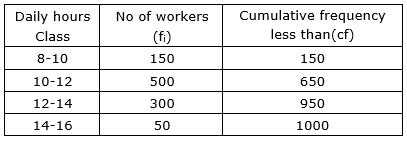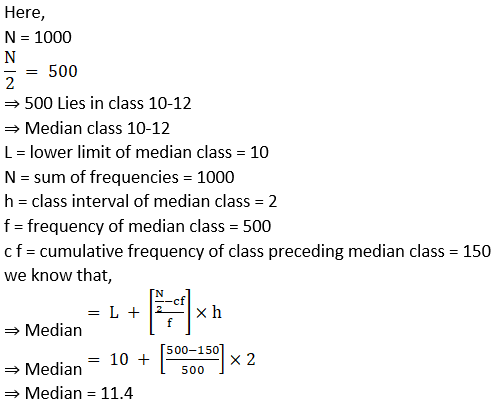2. The frequency distribution table shows the number of mango trees in a grove and their yield of mangoes. Find the median of data.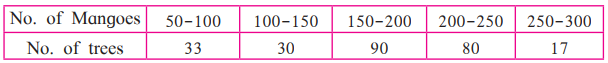Solution:

Using given data, we can construct a table.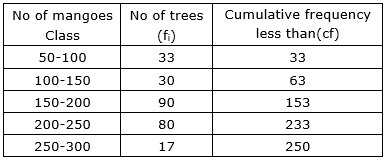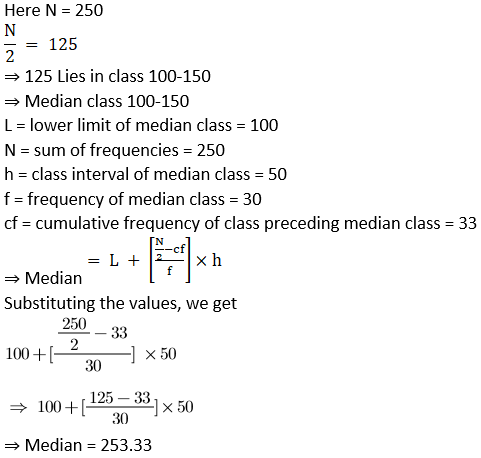Practice set 6.3 Page no: 149

1. The following table shows the information regarding the milk collected from farmers on a milk collection centre and the content of fat in the milk, measured by a lactometer. Find the mode of fat content.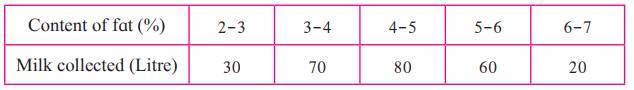Solution:

Using given data, we can construct a table.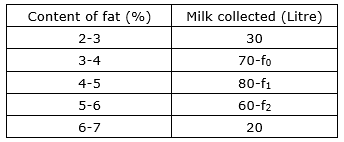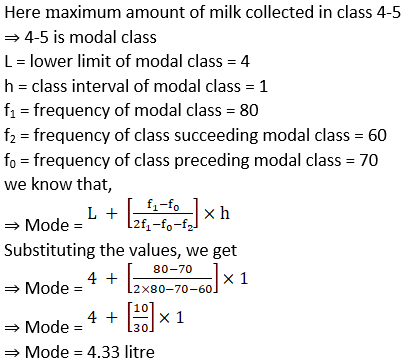2. Electricity used by some families is shown in the following table. Find the mode for use of electricity.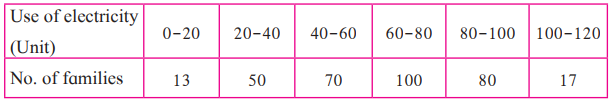Solution:

Using given data, we can construct a table.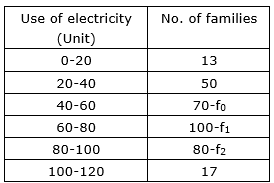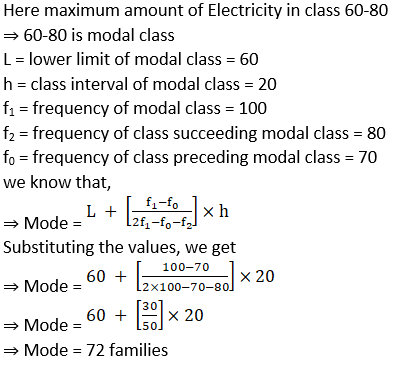Practice set 6.4 Page no: 153

1. Draw a histogram of the following data.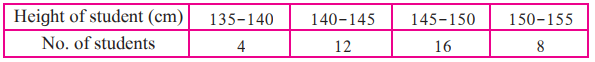Solution:

For the given data we can construct histogram which is given below.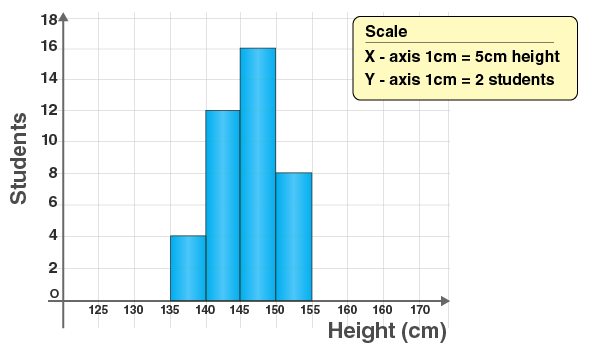2. The table below shows the yield of jowar per acre. Show the data by histogram.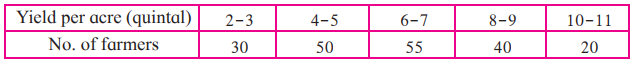Solution:

Given classes are not continuous, so let us construct a continuous class.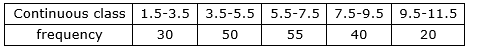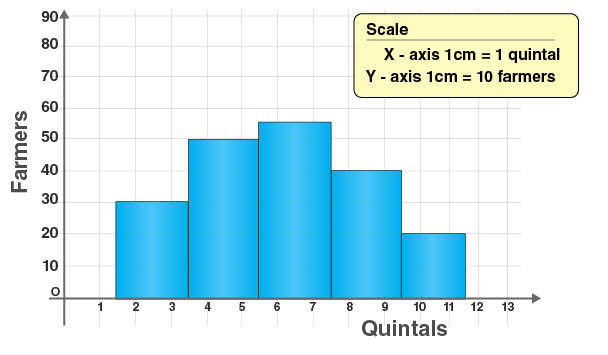Practice set 6.5 Page no: 156

1. Observe the following frequency polygon and write the answers of the questions below it.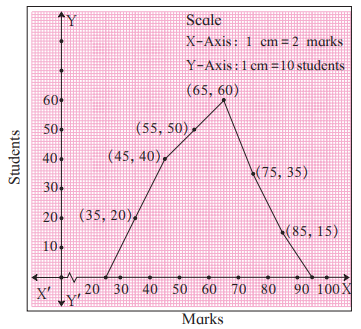(1) Which class has the maximum number of students?

Solution:

Class 60-70 has maximum number of students

(2) Write the classes having zero frequency.

Solution:

Class 20-30 and class 90-100 have zero frequency

(3) What is the class-mark of the class, having frequency of 50 students?

Solution:

Frequency 50 students is for class 50-60

Class mark for this class is 55

(4) Write the lower and upper-class limits of the class whose class mark is 85.

Solution:

Class mark is 85 for class 80-90

Lower limit = 80

Upper limit = 90

(5) How many students are in the class 80-90?

Solution:

There are 15 students in class 80-90

Practice set 6.6 Page no: 163

1. The age group and number of persons, who donated blood in a blood donation camp is given below. Draw a pie diagram from it.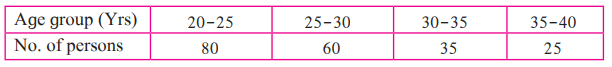Solution: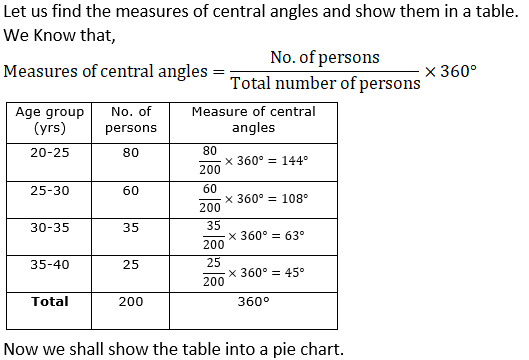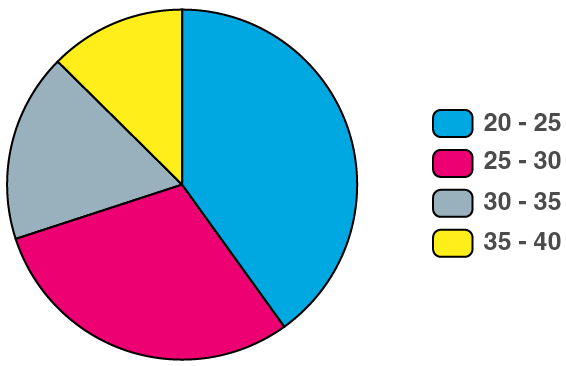2. The marks obtained by a student in different subjects are shown. Draw a pie diagram showing the information.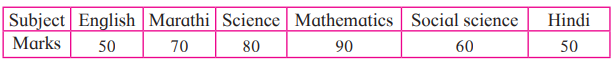Solution: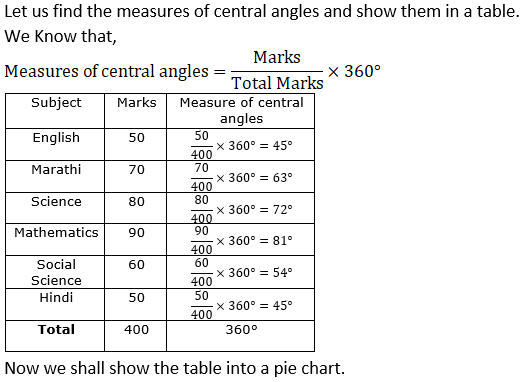Now we shall show the table into a pie chart.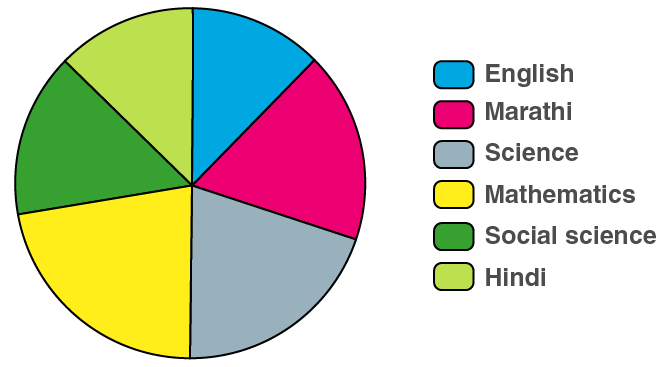3. In a tree plantation programme, the number of trees planted by students of different classes is given in the following table. Draw a pie diagram showing the information.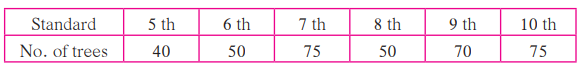Solution: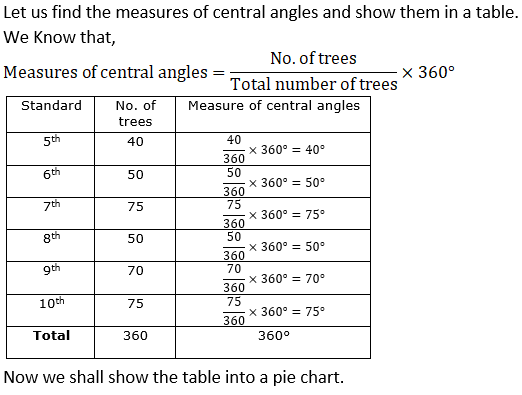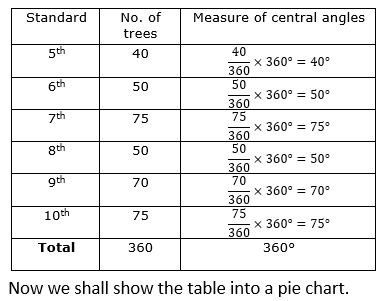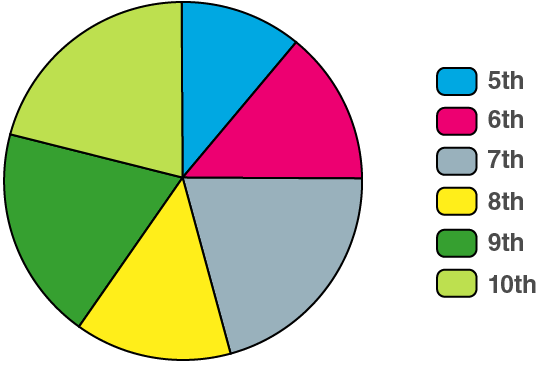Miscellaneous Problems – 6 Page no: 164

1. Find the correct answer from the alternatives given.

(1) The persons of O- blood group are 40%. The classification of persons based on blood groups is to be shown by a pie diagram. What should be the measures of angle for the persons of O- blood group?
A. 114°
B. 140°
C. 104°
D. 144°

Solution:

D. 144°

Explanation: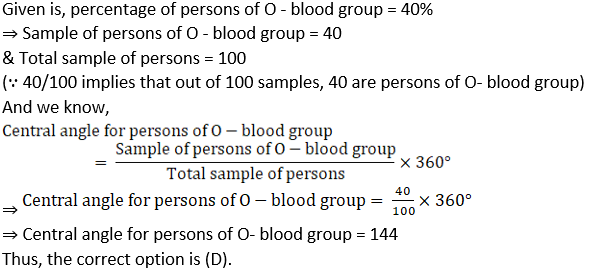(2) Different expenditures incurred on the construction of a building were shown by a pie diagram. The expenditure
Rs 45,000 on cement was shown by a sector of central angle of 75°. What was the total expenditure of the construction?
A. 2,16,000
B. 3,60,000
C. 4,50,000
D. 7,50,000

Solution:

A. 2,16,000

Explanation: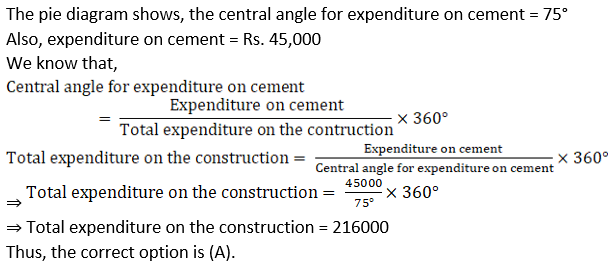(3) Cumulative frequencies in a grouped frequency table are useful to find . . .
A. Mean

B. Median
C. Mode
D. All of these

Solution:

B. Median

Explanation:

Median is the middle value of the set of ordered data. The position of the median is given by {(n + 1)/2}th value, where n is the number of values in a set of data.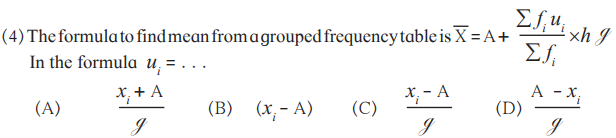Solution: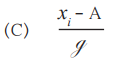Explanation:

Among the given option, (Xi – A)/g is the correct option.

Where Xi = values in the given data corresponding to ith position.

A = Assumed mean

g = class size

Thus, (C) is the correct option.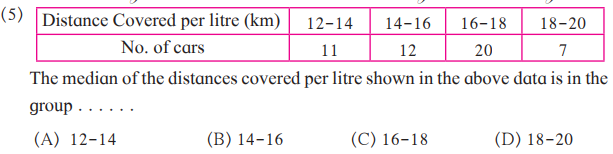The median of the distances covered per litre shown in the above data is in the group . . .. .
A. 12-14
B. 14-16
C. 16-18
D. 18-20

Solution:

C. 16-18

Explanation:

Here, (n/2)th = (50/2)th = 25th term

cf = 23 < 25 ⇒ Median class = 16-18

Median class is the next class of interval of cumulative frequency.

Thus, option (C) is correct.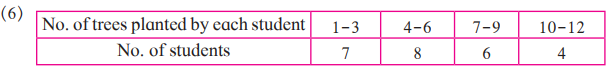The above data is to be shown by a frequency polygon. The coordinates of the points to show number of students in the class 4-6 are . . . .
A. (4, 8)
B. (3, 5)
C. (5, 8)
D. (8, 4)

Solution:

C. (5, 8)

Explanation: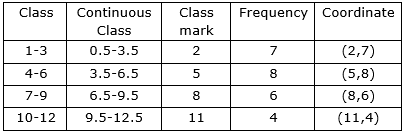So, the coordinates of the points to show number of students in the class 4-6 are (5,8).

Thus, option (C) is correct.

2. The following table shows the income of farmers in a grape season. Find the mean of their income.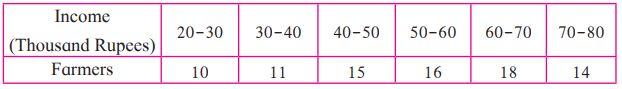Solution: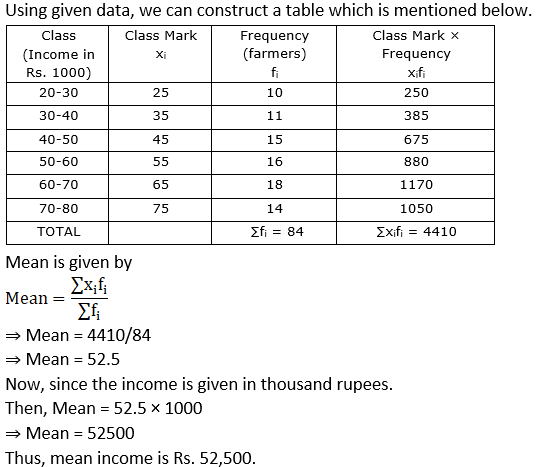Mean is given by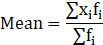⇒ Mean = 4410/84

⇒ Mean = 52.5

Now, since the income is given in thousand rupees.

Then, Mean = 52.5 × 1000

⇒ Mean = 52500

Thus, mean income is Rs. 52,500.

3. The loans sanctioned by a bank for construction of farm ponds are shown in the following table. Find the mean of the loans.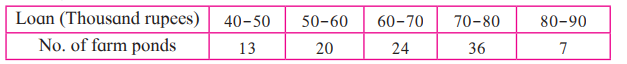Solution:

Using given data, we can construct a table which is mentioned below.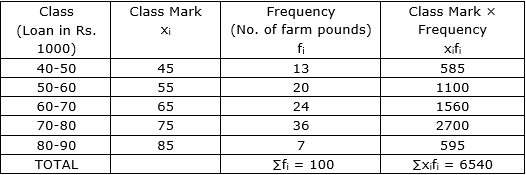Mean is given by⇒ Mean = 6540/100

⇒ Mean = 65.4

Now, since the loan is given in thousand rupees.

Then, Mean = 65.4 × 1000

⇒ Mean = 65400

Thus, mean loan is Rs. 65,400.

4. The weekly wages of 120 workers in a factory are shown in the following frequency distribution table. Find the mean of the weekly wages.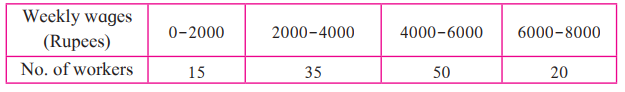Solution:

Using given data, we can construct a table which is mentioned below.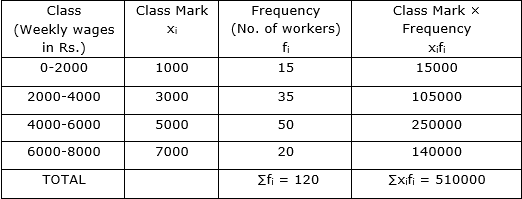Mean is given by⇒ Mean = 510000/120

⇒ Mean = 4250

Thus, mean weekly wages is Rs. 4250.

5. The following frequency distribution table shows the amount of aid given to 50 flood affected families. Find the mean of the amount of aid.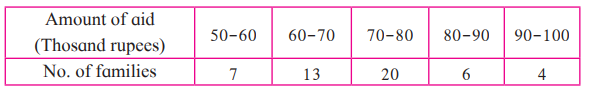Solution:

Using given data, we can construct a table which is mentioned below.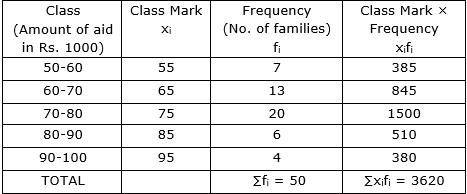Mean is given by⇒ Mean = 3620/50

⇒ Mean = 72.4

Now, since the amount of aid is given in thousand rupees.

Then, Mean = 72.4 × 1000

⇒ Mean = 72400

Thus, mean amount of aid is Rs. 72,400.

6. The distances covered by 250 public transport buses in a day is shown in the following frequency distribution table. Find the median of the distances.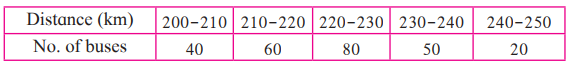Solution:

Let us prepare cumulative frequency table: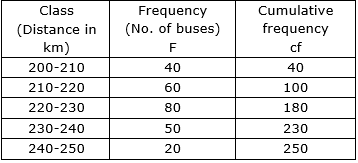Here, N = 250

N/2 = 250/2 = 125

Since, cumulative frequency 180 is just greater than 125. ⇒ median class = 220-230

Median is given by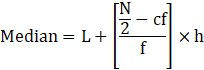Where l = lower limit of the median class

N = Sum of frequencies

h = class interval of the median class

cf = Cumulative frequency of the class preceding the median class

f = Frequency of the median class

Here, Median class = 220-230

L = 220

N/2 = 125

h = 10

cf = 100

f = 80

Putting all these values in the median formula, we get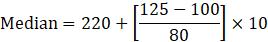⇒ Median = 220 + 250/80

⇒ Median = 220 + 3.125 = 223.125

Or Median is approximately 223.13 km

Thus, median of the distances is 223.13 km.

Statistics is a division of Maths that deals with the study of the collection, interpretation, arrangement, and exhibition of data. Mathematically, statistics is determined as a set of equations, used to analyse the data. Learn about the chapter Statistics at BYJU’S.

## Frequently Asked Questions on Maharashtra State Board Solutions for Class 10 Maths Part 1 Chapter 6 Statistics

### Will these Maharashtra Board Class 10 Solutions be useful to prepare for the exams?

Yes, these solutions can be very helpful. Students are highly recommended to practise these solutions after revising the subjects, as they set the basis for the questions that could get asked often in the board exams.

### How are these Maharashtra State Board Class 10 Maths Part 1 Chapter 6 Solutions helpful?

Students are encouraged to download these solutions and practise them. Then they can refer back to the solutions to analyse their performance. This will also help them to rectify their mistakes and to avoid making them during the board exams.

### Where can we find these Maharashtra Board Class 10 Solutions ?

Yes, we provide the solutions to download. We have also provided the solutions as a scrollable PDF and we have also mentioned the clickable link for the students to access. Meanwhile, the questions and the solutions  are also available online on our webpage.